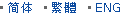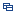| Recent Visit History | Join Audited Suppliers | Help | Region Guide
» » » » Nanjing Product List

151.

## Spherical Glass Optics Bi Convex Lens[Nov 20, 2020]

Bi Convex lens has a positive focal length, and with two outward surfaces. The two surfaces are with same radius of curvature, thereby minimizing spherical aberration. Some ...

152.

## N-Bk7 Optical Glass Double Convex Lens for Telescope[Nov 20, 2020]

Double Convex lens has a positive focal length, and with two outward surfaces. The two surfaces are with same radius of curvature, thereby minimizing spherical aberration. Some ...

153.

## Beam Expanding Double Concave Lens Optical Glass[Nov 20, 2020]

Double concave lenses have equal radius of curvature on both surfaces. The lenses are often used to expand light beams or to increase focal lengths in optical systems and devices, ...

154.

## Ar Coated Telescope Double Convex Glass Lens[Nov 20, 2020]

Double Convex lens has a positive focal length, and with two outward surfaces. The two surfaces are with same radius of curvature, thereby minimizing spherical aberration. Some ...

155.

## Laser Optics Precision Optical Glass Lens[Nov 20, 2020]

Meniscus lenses have one concave and one convex surface. For this lens type, the two curvatures are chosen to minimize the spherical aberration at one laser wavelength, hence ...

156.

## Calcium Fluoride Glass Bi Convex Lens[Nov 20, 2020]

Double convex lenses have two outward curved surfaces, normally the two surfaces have same radius of curvature, with finely polished. Bi convex lenses have positive focal length, ...

157.

## Factory Jgs1 Glass Bi Convex Collimating Lens[Nov 20, 2020]

Double convex lenses have two outward curved surfaces, normally the two surfaces have same radius of curvature, with finely polished. Bi convex lenses have positive focal length, ...

158.

## Optical Lens Plano Convex Lens Optical Glass[Nov 20, 2020]

Meniscus lenses have one concave and one convex surface. For this lens type, the two curvatures are chosen to minimize the spherical aberration at one laser wavelength, hence ...

159.

## Focusing Glass Lens Plano Convex Lens[Nov 20, 2020]

Plano convex lenses have one plano surface and one convex surface, they have a positive focal length. Plano convex lenses are widely used for focusing and collecting light in ...

160.

## Factory Silicon Glass Bi Convex Lens[Nov 20, 2020]

Double convex lenses have two outward curved surfaces, normally the two surfaces have same radius of curvature, with finely polished. Bi convex lenses have positive focal length, ...

161.

## Plano Convex Lens Illuminating Lens Optical Glass Lens[Nov 20, 2020]

Meniscus lenses have one concave and one convex surface. For this lens type, the two curvatures are chosen to minimize the spherical aberration at one laser wavelength, hence ...

162.

## Optical Glass Jgs1 Double Convex Lens[Nov 20, 2020]

Double convex lenses comprise two convex spherical surfaces, generally with the same radius of curvature. Bi convex lenses are used as magnifiers, condensing lenses and ...

163.

## Convex Concave Lens-Plano Concave Lens-Optical Glass Lens[Nov 20, 2020]

Meniscus lenses are designed to minimize spherical aberration. When used in combination with another lens, meniscus lenses will shorten the focal length and increase the NA of the ...

164.

## Factory Optical Glass Bi Convex Lens[Nov 20, 2020]

Double convex lenses have two outward curved surfaces, normally the two surfaces have same radius of curvature, with finely polished. Bi convex lenses have positive focal length, ...

165.

## Bi Concave Glass Objective Lens Ar Coated[Nov 20, 2020]

Bi concave lenses comprise of two inward equally curved surfaces. They are ideal for beam expansion, light projection and expanding the focal length of an optical system. Bi ...

Hot Region Index: ABCDEFGHIJKLMNPQRSTUWXYZ